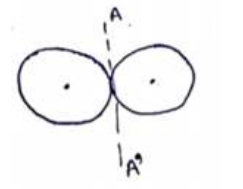# Solve the following :

Question:

Find the moment of inertia of a pair of spheres, each having a mass $\mathrm{m}$ and radius $\mathrm{r}$, kept in contact about the tangent passing through the point of contact.

Solution:By parallel axis theorem,

$I_{a x i s}=I_{C O M}+m r^{2}$

$I_{A A^{r}}=\left(\frac{2}{5} m r^{2}+m r^{2}\right) 2$

$I_{A A^{r}}=\frac{14}{5} m r^{2}$